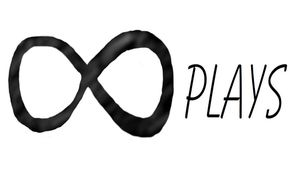By Bob

Sometimes it's handy to print a pegboard, here is a simple module that allows you to scale the board to make either a perfboard or a pegboard.

Here's the code:

``````/*[Pegboard/Perfboard]*/

show_pegs = false;
peg_length = 1;//[0:.01:25]
peg_shape = 50; //[3:1:50]
peg_diameter_1= .10;//[0:.01:20]
peg_diameter_2= .10;//[0:.01:20]
peg_offset= 0; //[-50:.01:50]
hole_number_X=10; //[1:1:100]
hole_number_Y=10; //[1:1:100]
hole_size = .35; //[0:.01:25.4]
hole_shape = 50; //[3:1:50]
hole_spacing = 2.54;//[2.54:.01:25.4]
board_thickness=1.2;//[.1:.01:25.4]

module board(){
translate([-hole_spacing/2,-hole_spacing/2,0])
cube(size=[(hole_number_X*hole_spacing)+4,(hole_number_Y*hole_spacing)+4,board_thickness],center=true);
}

module holes(){
for(a=[0:hole_number_X-1])
for(b=[0:hole_number_Y-1])
translate([(a-hole_number_X/2)*hole_spacing,(b-hole_number_Y/2)*hole_spacing,0])
cylinder(r1=peg_diameter_1+hole_size/2,r2=peg_diameter_2+hole_size/2,h=board_thickness+1,center=true,\$fn=hole_shape);
}

module pegs(){
for(c=[0:hole_number_X-1])
for(d=[0:hole_number_Y-1])
translate([(c-hole_number_X/2)*hole_spacing,(d-hole_number_Y/2)*hole_spacing,peg_length/2-(.5*board_thickness)+peg_offset])
cylinder(r1=peg_diameter_1,r2=peg_diameter_2,h=peg_length,center=true,\$fn=peg_shape);
}

difference(){
board();
holes();
}

if (show_pegs)
pegs();``````

it's pretty easy to customize the board to do multiple things, even make a bit holder:

I also added a way to change the length and diameter the pegs to hold thread spools or make the pegs cone shaped just for fun:

I will probably make a few more changes so the pegs can be adjusted to make holding pins for 2 part molds.

## Related ArticlesFollow